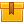# Series Circuits

## Prerequisites

Basic understanding of electrical circuits and a solid understanding of Ohm's law is recommended.

## Description

This is the third lesson in the iKNOW Electrical Theory for Troubleshooters Library. The lesson presents the operation of a series circuit and trains participants in the identification of simple schematic symbols used to represent components in a series circuit. The behavior of current, resistance, and current in a series circuit, and the used of Kirchhoff's Voltage Law to find total voltage are also covered.

## Objectives

Define a series circuit. Properly identify simple schematic symbols for a battery, switch, lamp, resistor and conductor. Describe how current and resistance behave in a series circuit. Describe how voltage behaves in a series circuit. Use Kirchhoff??s Voltage Law to find total voltage in a series circuit.

## ContentThis is the third lesson in the iKNOW™ Electrical Theory for
Troubleshooters Library. The lesson presents the operation of a series
circuit and trains participants in the identification of simple
schematic symbols used to represent components in a series circuit. The
behavior of current, resistance, and current in a series circuit, and
the used of Kirchhoff’s Voltage Law to find total voltage are also
covered.

•Series Circuits

0
0 Reviews